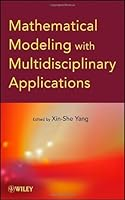# Mathematical Modeling with Multidisciplinary Applications1 Reviews
2013-01-14
592 pages

## Book Description

Features mathematical techniques and real-world processes with applications in diverse fields

with Multidisciplinary Applications details the interdisciplinary nature of mathematical modeling and numerical algorithms. The book combines a variety of applications from diverse fields to illustrate how the methods can be used to model processes, design new products, find solutions to challenging problems, and increase competitiveness in international markets.

Written by leading scholars and international experts in the field, the book presents new and emerging topics in areas including finance and , theoretical and applied mathematics, engineering and machine learning, physics, chemistry, ecology, and social science. In addition, the book thoroughly summarizes widely used mathematical and numerical methods in mathematical modeling and features:

• Diverse topics such as partial differential equations (PDEs), fractional calculus, inverse problems by ordinary differential equations (ODEs), semigroups, decision theory, risk analysis, Bayesian estimation, nonlinear PDEs in financial engineering, perturbation analysis, and dynamic system modeling
• Case studies and real-world applications that are widely used for current mathematical modeling courses, such as the green house effect and Stokes flow estimation
• Comprehensive coverage of a wide range of contemporary topics, such as theory, statistical models, and analytical solutions to numerical methods
• Examples, exercises with select solutions, and detailed references to the latest literature to solidify comprehensive learning
• New techniques and applications with balanced coverage of PDEs, discrete models, , fractional calculus, and more

Mathematical Modeling with Multidisciplinary Applications is an excellent book for courses on mathematical modeling and applied mathematics at the upper-undergraduate and graduate levels. The book also serves as a valuable reference for research scientists, mathematicians, and engineers who would like to develop further insights into essential mathematical tools.

Part I. Introduction and Foundations
1. Differential Equations 3
2. Mathematical Modelling 23
3. Numerical Methods: An Introduction 45
4. Teaching Mathematical Modelling 57

Part II. Mathematical Modelling with Multidisciplinary Applications
5. Industrial Mathematics with Applications 83
6. Data Analysis in Economics 123
7. Inverse Problems in ODEs 151
8. Estimation of Model Parameters 169
9. Parabolic PDE in Financial Engineering 191
10. Decision Modeling in Supply Chain Management 229
11. Modelling Pricing Weather Derivatives 257
12. Decision Theory in Social Sciences 285
13. Fractals, with applications to signal and image modeling 307
14. Efficient Numerical Methods for Singularly Perturbed Differential Equations 329

15. Fractional Calculus and its Applications 357
16. Goal Programming Model 397
17. Decision Theory and Game Theory 421
18. Control Problems on Differential Equations 449
19. Markoc-jump stochastic models 473

## Book Details

• Title: Mathematical Modeling with Multidisciplinary Applications
• Length: 592 pages
• Edition: 1
• Language: English
• Publisher:
• Publication Date: 2013-01-14
• ISBN-10: 1118294416
• ISBN-13: 9781118294413